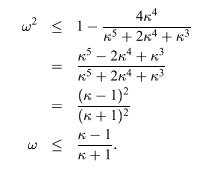## Linear regression in a few equations and optimization with deepest gradient descent

Linear regression in a few equations and optimization with steepest gradient descent

The linear regression problem, put simply, is solving $Xb = y$. In all practical situations, there is no solution, so we minimize the error $(Xb - y)^T(Xb - y)$, which is called the least squares method.

\begin{align*} 2g(b)&= (Xb - y)^T(Xb - y) \\ &= b^TX^TXb - 2y^TXb + y^Ty \\ g(b) &= \frac{1}{2}b^TX^TXb - y^TXb + \frac{1}{2}y^Ty \\ &= \frac{1}{2}b^TAb - d^Tb + c \end{align*}

here $A$ is the symmetric matrix $X^TX$, and $d = X^Ty$, and $b$ is the coefficient vector we will estimate.

Here is a direct way of doing it: To minimize $g(b)$, we set $\partial g / \partial b = 0$, which means:

\begin{align*} 2X^TXb - 2X^Ty &= 0 \\ b &= (X^TX)^{-1}X^Ty \\ \end{align*}

We don't really need steepest descent here, but let's do an exercise. The building blocks of the algorithm are these (with $b_{(0)}$ being a random vector, the starting point does not really matter):

\begin{align} b_{(i)} &= b_{(i-1)} + \alpha_{(i-1)} r_{(i-1)} \\ r_{(i)} &= d - Ab_{(i)} \\ \alpha_{(i)} &= \frac{r_{(i)}^T r_{(i)}}{r_{(i)}^T A r_{(i)}} \\ \end{align}

From equation (1), if we multiply both sides by $-A$ and add $b$, we have

\begin{align} r_{(i)} = r_{(i-1)} - \alpha_{(i-1)}Ar_{(i-1)} \end{align}

which eliminates one of the matrix multiplications. So the first iteration is:

\begin{align*} b_{(0)} &= 0 \\ r_{(0)} &= d - Ab_{(0)} \\ \alpha_{(0)} &= \frac{r_{(0)}^T r_{(0)}}{r_{(0)}^T A r_{(0)}} \\ \end{align*}

This does involve two matrix multiplications. $Ab_{(0)}$ and $Ar_{(0)}$. After that, things gets simpler:

\begin{align} b_{(i)} &= b_{(i-1)} + \alpha_{(i-1)} r_{(i-1)} \\ r_{(i)} &= r_{(i-1)} - \alpha_{(i-1)}Ar_{(i-1)} \\ \alpha_{(i)} &= \frac{r_{(i)}^T r_{(i)}}{r_{(i)}^T A r_{(i)}} \\ \end{align}

So, let's get concrete and list the first iterations in the steepest gradient descent algorithm:

\begin{align*} \text{Iteration 1} \\ b_{(0)} &= 0 \\ r_{(0)} &= d - Ab_{(0)} \\ \alpha_{(0)} &= \frac{r_{(0)}^T r_{(0)}}{r_{(0)}^T A r_{(0)}} \\ \end{align*} \begin{align*} \text{Iteration 2} \\ b_{(1)} &= b_{(0)} + \alpha_{(0)} r_{(0)} \\ r_{(1)} &= r_{(0)} - \alpha_{0}Ar_{(0)} \\ \alpha_{(1)} &= \frac{r_{(1)}^T r_{(1)}}{r_{(1)}^T A r_{(1)}} \\ \end{align*} \begin{align*} \text{Iteration 3} \\ b_{(2)} &= b_{(1)} + \alpha_{(1)} r_{(1)} \\ r_{(2)} &= r_{(1)} - \alpha_{1}Ar_{(1)} \\ \alpha_{(2)} &= \frac{r_{(2)}^T r_{(2)}}{r_{(2)}^T A r_{(2)}} \\ \vdots \end{align*}

The order of variables being computed:

Note the diagonal lines indicate how matrix multplication results are reused, thus reducing computational burden by half.

### One step convergence

As a reminder:

\begin{align} b_{(i)} &= b_{(i-1)} + \alpha_{(i-1)} r_{(i-1)} \\ r_{(i)} &= r_{(i-1)} - \alpha_{(i-1)}Ar_{(i-1)} \\ \alpha_{(i)} &= \frac{r_{(i)}^T r_{(i)}}{r_{(i)}^T A r_{(i)}} \\ \end{align}

Remember also the gradient is $Ab_{(i)} - d$, at the optimum, the gradient is equal to zero. The residual is the additive inverse of the gradient, i.e. $$r_{(i)} = d - Ab_{(i)}$$. And $$e_{(i)} = b_{(i)} - b$$, so $$r_{(i)} = -Ae_{(i)}$$

From equation (8), if we subtract $b$ from both sides, we get

\begin{align} e_{(i)} &= e_{(i-1)} + \alpha_{(i-1)} r_{(i-1)} \\ &= e_{(i-1)} + \frac{r_{(i-1)}^T r_{(i-1)}}{r_{(i-1)}^T A r_{(i-1)}} r_{(i-1)} \\ \end{align}

Now we are going to consider two cases where the steepest gradient method will reach the optimum in one step.

First suppose $e_{(i)}$ is an eigenvector of $A$ with eigenvalue $\lambda$. Then $$r_{(i-1)} = -Ae_{(i-1)} = -\lambda e_{(i-1)}$$ and $r_{(i-1)}$ is also an eigenvector with the same eigenvalue. so

\begin{align} e_{(i)} &= e_{(i-1)} + \frac{r_{(i-1)}^T r_{(i-1)}}{r_{(i-1)}^T A r_{(i-1)}} r_{(i-1)} \\ &= e_{(i-1)} + \frac{r_{(i-1)}^T r_{(i-1)}}{\lambda r_{(i-1)}^T r_{(i-1)}} r_{(i-1)} \\ &= e_{(i-1)} + \frac{1}{\lambda} r_{(i-1)} \\ &= 0 \\ \end{align}

You see, if we take the step size $\alpha = 1/\lambda$, we make $e = 0$ in one step.

Secondly, suppose $A$ has $n$ independent eigenvectors, in other words, its eigenspace has n dimensions, then these eigenvectors can be orthogonalized, i.e. be made orthogonal to each other, and each has norm 1. Then each error vector can be decomposed into this eigenspace.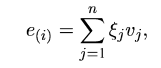Other relevant variables can also be rewritten in terms of those eigenvectors: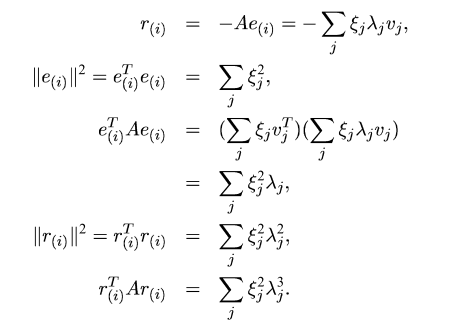Then the iteration of error goes like this: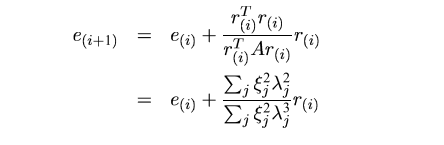If all eigenvalues are the same, then again:

\begin{align*} r_{(i)} &= - \lambda e_{(i)} \\ e_{(i)} &= e_{(i-1)} + \frac{1}{\lambda} r_{(i-1)} \\ &= 0 \\ \end{align*}

### General convergence

For general convergence, we observe for a quadratic form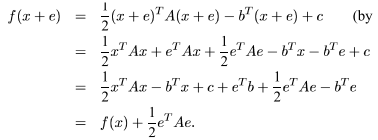\begin{align*} g(b+e) &= \frac{1}{2}b^TX^TXb - y^TXb + \frac{1}{2}y^Ty \\ &= \frac{1}{2}b^TAb - d^Tb + c \\ &= g(b) + \frac{1}{2}e^TAe \end{align*}

The idea is, if we want to arrive (nearly) at the true value of $b$, we definitely need to minimize $e^TAe$. So we define a something new, $\sqrt{e^Te}$ and call it the energy norm. And with this norm we have: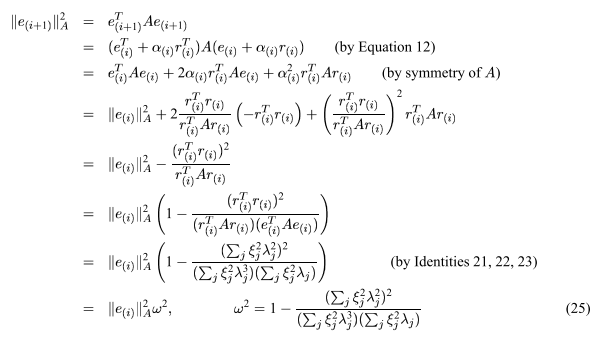Now the speed of convergence depends on $\omega^2$. Let $\kappa = \lambda_1 / \lambda_2 \ge 1$ (spectral condition number), and $\mu = \xi_2 / \xi_1$.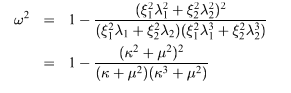Relation between $\omega^2, \; \kappa, \; \mu$ is visualized here: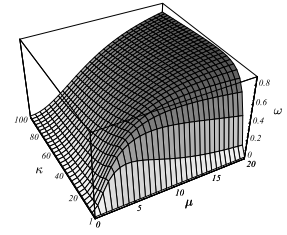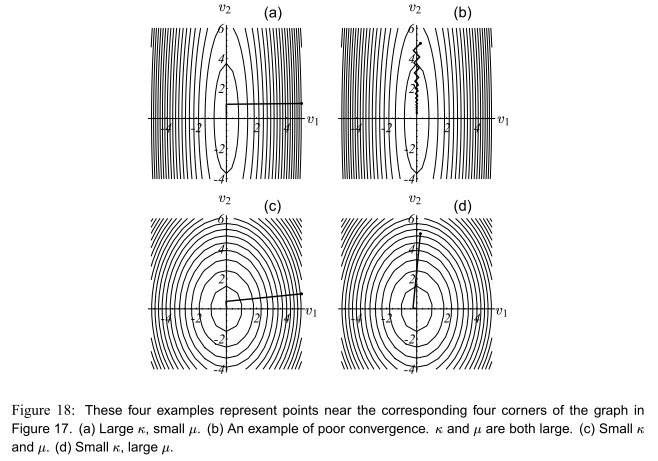$\kappa \ge 1$ and $\mu^2 \ge 0$. We had better see $\mu^2$ as a whole here. Consider its extreme values here, when $\mu^2 = 0$ or $\mu^2 = \infty$, we have $\omega^2 = 0$, which is the minimum value of $\omega^2$. It's a simple calculus exercise to find out that $\omega^2$ takes it maximum at $\mu^2 = \kappa^2$. Using this we get: